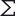# Statistics

This category contains 14 nodes.

##### Cronbach Alpha

Computes the Cronbach Alpha for all numerical columns based on their variance.

##### Crosstab (local)

Creates a cross-tabulation (also referred as contingency table or cross-tab).

##### Linear Correlation

Computes correlation coefficients for pairs of numeric or nominal columns.

##### Numeric Outliers

Detects and handles outliers for all numerical columns.

##### Numeric Outliers (Apply)Streamable

Handles outliers for all numerical columns according to the provided model.

##### Rank Correlation

Computes correlation coefficients for pairs of columns, based on the sorting of its values only.

##### Rank CorrelationDeprecated

Computes correlation coefficients for pairs of columns, based on the sorting of its values only.

##### Standardized Cronbach Alpha

Calculate the standardized Cronbach Alpha, a general measure for internal consistency of the data.

##### Statistics

Calculates statistic moments with their distributions as histograms and counts nominal values and their occurrences across all columns.